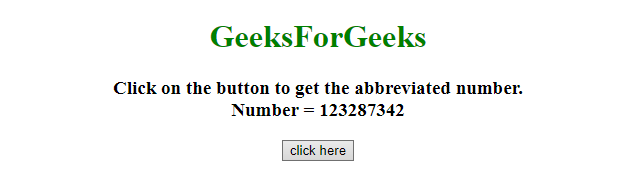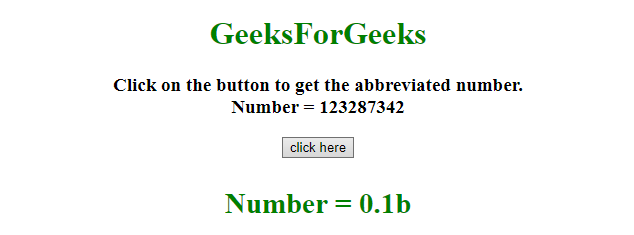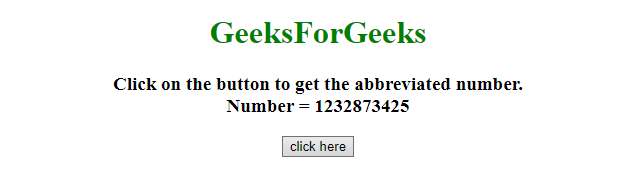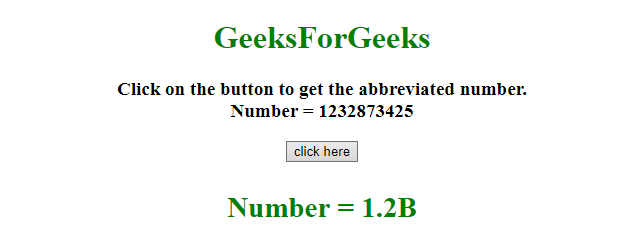# How to convert long number into abbreviated string in JavaScript ?

Given a long number and the task is to convert it to the abbreviated string(eg.. 1234 to 1.2k). Here 2 approaches are discussed with the help of javaScript.

Approach 1:

• Get the characters in an array(ar = [“”, “k”, “m”, “b”])
• Divide the length of the number by 3 and get value in var(sNum).
• If the sNum != 0 then calculate the precised value of the number by dividing it by 1000^sNum.
• Append the character at index = sNum in the array, to the precised value to finally get the abbreviated number.

Example 1: This example implements the above approach.

 ` ` `<``html``> ` ` `  `<``head``> ` `    ``<``title``> ` `        ``Convert long number into abbreviated string. ` `    `` ` `    ``<``script` `src``= ` `"https://ajax.googleapis.com/ajax/libs/jquery/3.4.0/jquery.min.js"``> ` `    `` ` ` ` ` `  `<``body` `style``=``"text-align:center;"``> ` `    ``<``h1` `style``=``"color:green;"``>   ` `            ``GeeksForGeeks   ` `        `` ` `    ``<``p` `id``=``"GFG_UP"``> ` `    `` ` `    ``<``button` `onclick``=``"GFG_Fun();"``> ` `        ``click here ` `    `` ` `    ``<``p` `id``=``"GFG_DOWN"` `style``=``"color: green;"``> ` `    `` ` `    ``<``script``> ` `        ``var up = document.getElementById('GFG_UP'); ` `        ``var down = document.getElementById('GFG_DOWN'); ` `        ``var n = 123287342; ` `        ``up.innerHTML = ` `"Click on the button to get the abbreviated number.<``br``>Number = " ` `        ``+ n; ` ` `  `        ``function convrt(val) { ` ` `  `            ``// thousands, millions, billions etc.. ` `            ``var s = ["", "k", "m", "b", "t"]; ` ` `  `            ``// dividing the value by 3. ` `            ``var sNum = Math.floor(("" + val).length / 3); ` ` `  `            ``// calculating the precised value. ` `            ``var sVal = parseFloat(( ` `              ``sNum != 0 ? (val / Math.pow(1000, sNum)) : val).toPrecision(2)); ` `           `  `            ``if (sVal % 1 != 0) { ` `                ``sVal = sVal.toFixed(1); ` `            ``} ` ` `  `            ``// appending the letter to precised val. ` `            ``return sVal + s[sNum]; ` `        ``} ` ` `  `        ``function GFG_Fun() { ` `            ``\$('#GFG_DOWN').html('Number = ' + convrt(n)); ` `        ``} ` `    `` ` ` ` ` `  ` `

Output:

• Before clicking on the button:• After clicking on the button:Approach 2:

• Check if the number is less than 1e3, if it is then return the number as it is.
• If the number is greater than or equal to 1e3 and less than 1e6 then remove the last three digits and append character ‘K’ to it.
• If the number is greater than or equal to 1e6 and less than 1e9 then remove the last six digits and append character ‘M’ to it.
• If the number is greater than or equal to 1e9 and less than 1e12 then remove the last nine digits and append character ‘B’ to it.
• If the number is greater than or equal to 1e12 remove the last 12 digits and append character ‘T’ to it.

Example 2: This example implements the above approach.

 ` ` `<``html``> ` ` `  `<``head``> ` `    ``<``title``> ` `        ``Convert long number into abbreviated string. ` `    `` ` `    ``<``script` `src``= ` `"https://ajax.googleapis.com/ajax/libs/jquery/3.4.0/jquery.min.js"``> ` `    `` ` ` ` ` `  `<``body` `style``=``"text-align:center;"``> ` `    ``<``h1` `style``=``"color:green;"``>   ` `            ``GeeksForGeeks   ` `        `` ` `    ``<``p` `id``=``"GFG_UP"``> ` `    `` ` `    ``<``button` `onclick``=``"GFG_Fun();"``> ` `        ``click here ` `    `` ` `    ``<``p` `id``=``"GFG_DOWN"` `style``=``"color: green;"``> ` `    `` ` `    ``<``script``> ` `        ``var up = document.getElementById('GFG_UP'); ` `        ``var down = document.getElementById('GFG_DOWN'); ` `        ``var n = 1232873425; ` `        ``up.innerHTML =  ` `          ``"Click on the button to get the abbreviated number.<``br``>Number = " ` `        ``+ n; ` `        ``const convrt = n => { ` `            ``if (n < ``1e3``) return n; ` `            ``if (n >= 1e3 && n < ``1e6``) return +(n / 1e3).toFixed(1) + "K"; ` `            ``if (n >= 1e6 && n < ``1e9``) return +(n / 1e6).toFixed(1) + "M"; ` `            ``if (n >= 1e9 && n < ``1e12``) return +(n / 1e9).toFixed(1) + "B"; ` `            ``if (n >= 1e12) return +(n / 1e12).toFixed(1) + "T"; ` `        ``}; ` ` `  `        ``function GFG_Fun() { ` `            ``\$('#GFG_DOWN').html('Number = ' + convrt(n)); ` `        ``} ` `    `` ` ` ` ` `  ` `

Output:

• Before clicking on the button:• After clicking on the button:My Personal Notes arrow_drop_upCheck out this Author's contributed articles.

If you like GeeksforGeeks and would like to contribute, you can also write an article using contribute.geeksforgeeks.org or mail your article to contribute@geeksforgeeks.org. See your article appearing on the GeeksforGeeks main page and help other Geeks.

Please Improve this article if you find anything incorrect by clicking on the "Improve Article" button below.